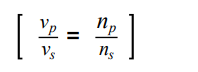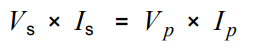# AQA GCSE Physics Transformer Focus Questions #gcserevision #physics #gcsephysics

1. Draw a labelled diagram of a transformer.
2. Why is iron used for its core?
3. Copy and complete the following: The _________ of the potential ___________ across the primary and secondary coils of a transformer Vp and Vs depends on the ___________ of the number of turns on each coil, np and ns .
4. What do p and s represent in question 63?
5. Have a look at this equation?6. What does the vo stand for?
7. Rearrange the equation four ways so that each term is the subject of the equation.
8. What is the difference between a step up and a step-down transformer?
9. If a transformer was 100% efficient then what could you say about the power in and power out?
10. What is the equation to calculate electrical power in terms of current and potential difference?
11. What is the unit of electrical power?
12. Look at this equation.13. Can you explain what this means? What is on the left-hand side and what is on the right-hand side of the equation?
14. If the potential difference is stepped up what happens to the current in the secondary coil? Why is the useful in terms of the transmission of power across the power lines that make up the national grid?
15. If the potential difference is stepped down what happens to the current in the secondary coil?
16. Why is an alternating current used across the primary coil?
17. How hie an alternating current induced across the secondary coil? Explain this in terms of a changing magnetic field.